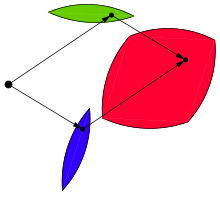The red figure is the Minkowski sum of blue and green figures.

In geometry, the Minkowski sum (also known as dilation) of two sets of position vectors A and B in Euclidean space is formed by adding each vector in A to each vector in B, i.e., the setAnalogously, the Minkowski difference (or geometric difference) is defined asIt is important to note that in general. For instance, in a one-dimensional caseandthe Minkowski difference, whereasThe correct formula connecting Minkowski sum and difference is as follows (herestands for the complement of):In a two-dimensional case, Minkowski difference is closely related to erosion (morphology) in image processing.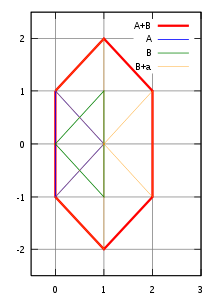Minkowski sum A + B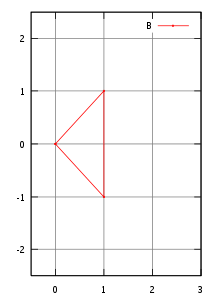B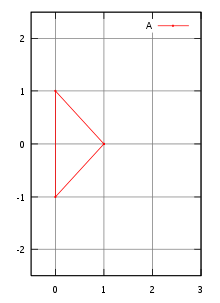A

The concept is named for Hermann Minkowski.

## Example

For example, if we have two sets A and B, each consisting of three position vectors (informally, three points), representing the vertices of two triangles in, with coordinates

A = {(1, 0), (0, 1), (0, −1)}

and

B = {(0, 0), (1, 1), (1, −1)} ,

then the Minkowski sum is
A + B = {(1, 0), (2, 1), (2, −1), (0, 1), (1, 2), (1, 0), (0, −1), (1, 0), (1, −2)}, which looks like a hexagon, with three 'repeated' points at (1, 0).

For Minkowski addition, the zero set {0}, containing only the zero vector 0, is an identity element: For every subset S, of a vector space

S + {0} = S;

The empty set is important in Minkowski addition, because the empty set annihilates every other subset: for every subset, S, of a vector space, its sum with the empty set is empty: S +=.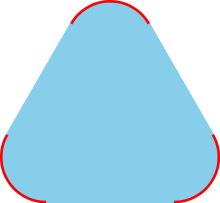In the convex hull of the red set, each blue point is a convex combination of some red points.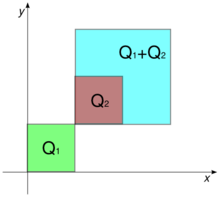Minkowski addition of sets. The sum of the squares Q1=[0,1]2 and Q2=[1,2]2 is the square Q1+Q2=[1,3]2.

## Convex hulls of Minkowski sums

Minkowski addition behaves well with respect to the operation of taking convex hulls, as shown by the following proposition:

• For all non-empty subsets S1 and S2 of a real vector-space, the convex hull of their Minkowski sum is the Minkowski sum of their convex hulls
Conv(S1 + S2) = Conv(S1) + Conv(S2).

This result holds more generally for each finite collection of non-empty sets

Conv(∑Sn) = ∑Conv(Sn).

In mathematical terminology, the operations of Minkowski summation and of forming convex hulls are commuting operations.

If S is a convex set then alsois a convex set; furthermorefor every.

Conversely, if this "distributive property" holds for all non-negative real numbers,, then the set is convex.

The figure shows an example of a non-convex set for which A + A ⊋ 2A.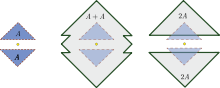An example of a non-convex set such that A + A ≠ 2A

An example in 1 dimension is: B=[1,2]∪[4,5]. It can be easily calculated that 2B=[2,4]∪[8,10] but B+B=[2,4]∪[5,7]∪[8,10], hence again B+B ⊋ 2B.

Minkowski sums act linearly on the perimeter of two-dimensional convex bodies: the perimeter of the sum equals the sum of perimeters. Additionally, if K is (the interior of) a curve of constant width, then the Minkowski sum of K and of its 180° rotation is a disk. These two facts can be combined to give a short proof of Barbier's theorem on the perimeter of curves of constant width.

## Applications

Minkowski addition plays a central role in mathematical morphology. It arises in the brush-and-stroke paradigm of 2D computer graphics (with various uses, notably by Donald E. Knuth in Metafont), and as the solid sweep operation of 3D computer graphics.

### Motion planning

Minkowski sums are used in motion planning of an object among obstacles. They are used for the computation of the configuration space, which is the set of all admissible positions of the object. In the simple model of translational motion of an object in the plane, where the position of an object may be uniquely specified by the position of a fixed point of this object, the configuration space are the Minkowski sum of the set of obstacles and the movable object placed at the origin and rotated 180 degrees.

### Numerical control (NC) machining

In numerical control machining, the programming of the NC tool exploits the fact that the Minkowski sum of the cutting piece with its trajectory gives the shape of the cut in the material.

### 3d Solid Modeling

In OpenSCAD Minkowski sums are used to outline a shape with another shape creating a composite of both shapes.

### Aggregation Theory

Minkowski sums are also frequently used in aggregation theory when individual objects to be aggregated are characterized via sets.

## Algorithms for computing Minkowski sums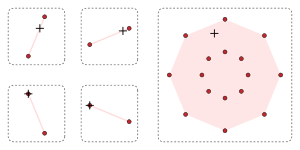Minkowski addition and convex hulls. The sixteen dark-red points (on the right) form the Minkowski sum of the four non-convex sets (on the left), each of which consists of a pair of red points. Their convex hulls (shaded pink) contain plus-signs (+): The right plus-sign is the sum of the left plus-signs.

### Planar case

#### Two convex polygons in the plane

For two convex polygons P and Q in the plane with m and n vertices, their Minkowski sum is a convex polygon with at most m + n vertices and may be computed in time O (m + n) by a very simple procedure, which may be informally described as follows. Assume that the edges of a polygon are given and the direction, say, counterclockwise, along the polygon boundary. Then it is easily seen that these edges of the convex polygon are ordered by polar angle. Let us merge the ordered sequences of the directed edges from P and Q into a single ordered sequence S. Imagine that these edges are solid arrows which can be moved freely while keeping them parallel to their original direction. Assemble these arrows in the order of the sequence S by attaching the tail of the next arrow to the head of the previous arrow. It turns out that the resulting polygonal chain will in fact be a convex polygon which is the Minkowski sum of P and Q.

#### Other

If one polygon is convex and another one is not, the complexity of their Minkowski sum is O(nm). If both of them are nonconvex, their Minkowski sum complexity is O((mn)2).

## Essential Minkowski sum

There is also a notion of the essential Minkowski sum +e of two subsets of Euclidean space. Note that the usual Minkowski sum can be written asThus, the essential Minkowski sum is defined bywhere μ denotes the n-dimensional Lebesgue measure. The reason for the term "essential" is the following property of indicator functions: whileit can be seen thatwhere "esssup" denotes the essential supremum.

## Notes

1. Hadwiger, Hugo (1950), Minkowskische addition und subtraktion beliebiger punktmengen und die theoreme von Erhard Schmidt, 53 (3), pp. 210–218
2. Theorem 3 (pages 562–563): Krein, M.; Šmulian, V. (1940). "On regularly convex sets in the space conjugate to a Banach space". Annals of Mathematics (2), Second series. 41. pp. 556–583. doi:10.2307/1968735. JSTOR 1968735. MR 2009.
3. For the commutativity of Minkowski addition and convexification, see Theorem 1.1.2 (pages 2–3) in Schneider; this reference discusses much of the literature on the convex hulls of Minkowski sumsets in its "Chapter 3 Minkowski addition" (pages 126–196): Schneider, Rolf (1993). Convex bodies: The Brunn–Minkowski theory. Encyclopedia of mathematics and its applications. 44. Cambridge: Cambridge University Press. pp. xiv+490. ISBN 0-521-35220-7. MR 1216521.
4. Chapter 1: Schneider, Rolf (1993). Convex bodies: The Brunn–Minkowski theory. Encyclopedia of mathematics and its applications. 44. Cambridge: Cambridge University Press. pp. xiv+490. ISBN 0-521-35220-7. MR 1216521.
5. Zelenyuk, V. (2015) "Aggregation of scale efficiency," European Journal of Operational Research, 240:1, pp 269-277.
6. Mayer, A. and Zelenyuk, V. (2014) "Aggregation of Malmquist productivity indexes allowing for reallocation of resources," European Journal of Operational Research, 238:3, pp 774-785